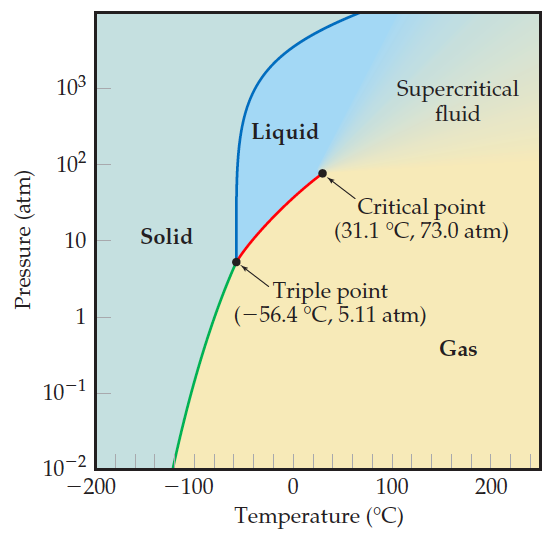# Problem: Referring to Figure 11.29, describe the phase changes (and the temperatures at which they occur) when CO2 is heated from –80 to –20°C at a constant pressure of 6 atm.

91% (30 ratings)
###### Problem Details

Referring to Figure 11.29, describe the phase changes (and the temperatures at which they occur) when CO2 is heated from –80 to –20°C at a constant pressure of 6 atm.Frequently Asked Questions

What scientific concept do you need to know in order to solve this problem?

Our tutors have indicated that to solve this problem you will need to apply the Phase Diagram concept. You can view video lessons to learn Phase Diagram. Or if you need more Phase Diagram practice, you can also practice Phase Diagram practice problems.